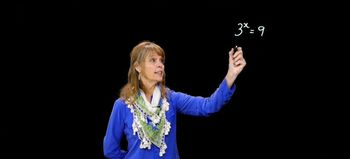Start typing, then use the up and down arrows to select an option from the list.
8:12 minutes
Problem 25
Textbook Question

# Solve each exponential equation in Exercises 23–48. Express the solution set in terms of natural logarithms or common logarithms. Then use a calculator to obtain a decimal approximation, correct to two decimal places, for the solution. e^x=5.7Verified Solution
This video solution was recommended by our tutors as helpful for the problem above.
62views